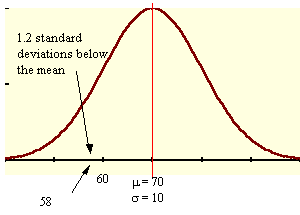### CFA Practice Question

There are 985 practice questions for this topic.

### CFA Practice Question

For x, a normal random variable with mean = 70 and standard deviation = 10, what x-score is 1.2 standard deviations below the mean?

A. 82
B. 58
C. 68.8

The mean minus 1.2 standard deviations is 70 - 1.2(10) = 70 - 12 = 58.User Comment
bentang can someone explain the formula?
bobert The highest point on the normal distribution is the mean, therefore the middle. 1 standard deviation is going to be +/- 10. The question asks for 1.2 standard deviations BELOW the mean. Because it is below we are going to be subtracting, and since 1 standard deviation = 10, 1.2 = 12. This leaves us with 70 - 12 = 58.
JKiro 1st note: this is a normal distribution
mean of 70 & std dev. of 10--> 1 std dev. (given)
looking for value of x to result in 1.2 std dev
(1.2/1)(10) = 12 is the resultant shift from the mean (positive or negative) given a 1.2 std dev.
for negavite shift (below): x = 70 - 12 --> 58
for positive shift (above): x = 70 + 12 --> 82
the question asked for the value of x (from the mean) below: 58
tschorsch I think that the distribution is irrelevant for this question. 1.2 std devs below the mean should always be mean - 1.2 x stddev
peteSP mean / 1.2 = 58
2014 Pete what if they ask above 58 would be wrong